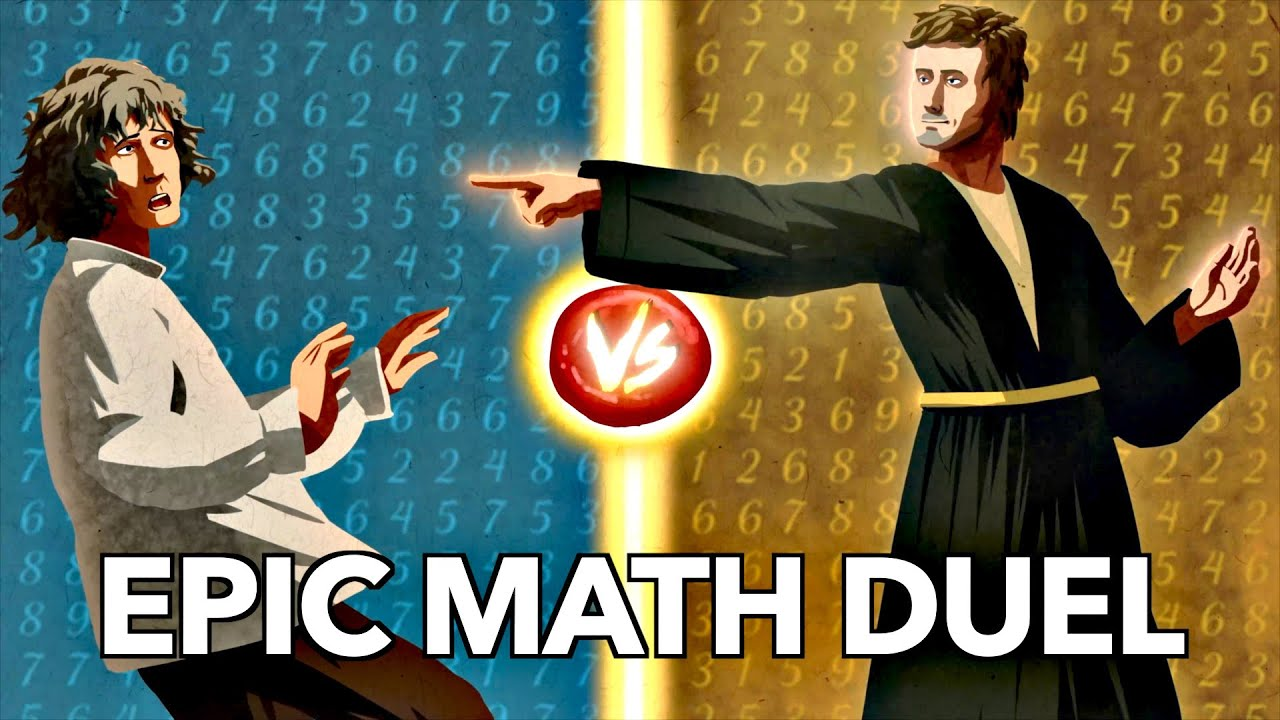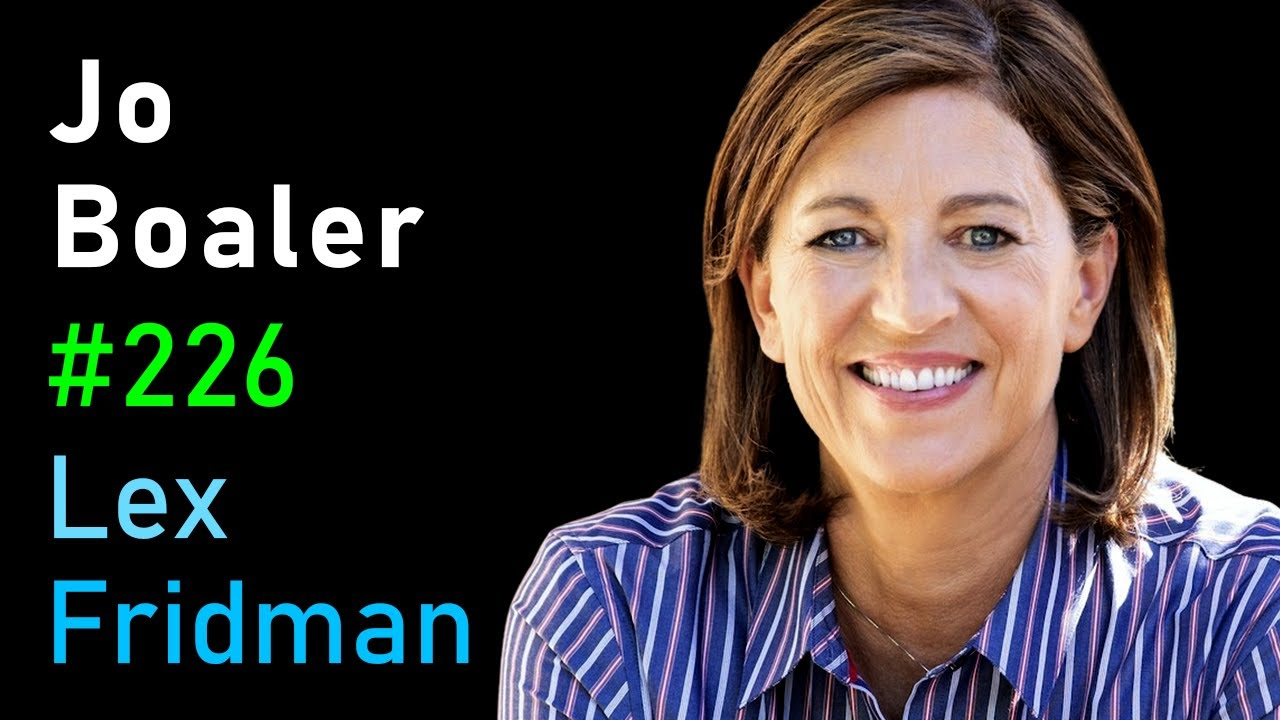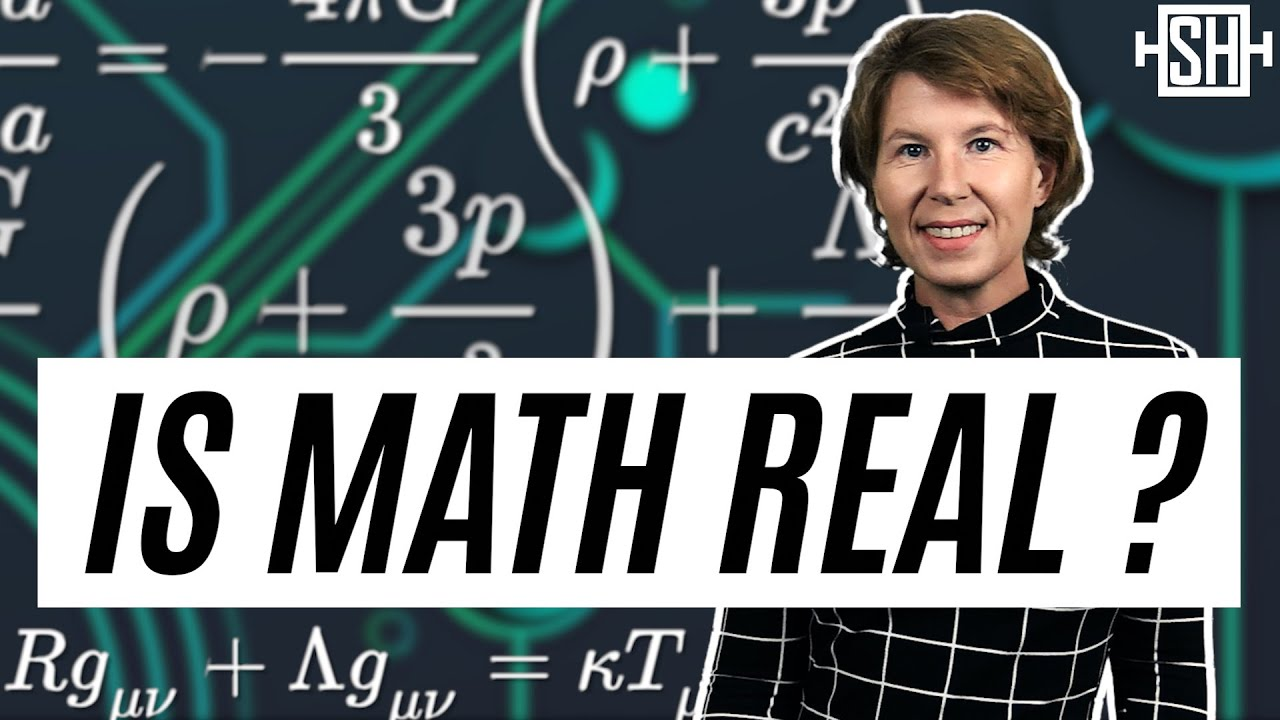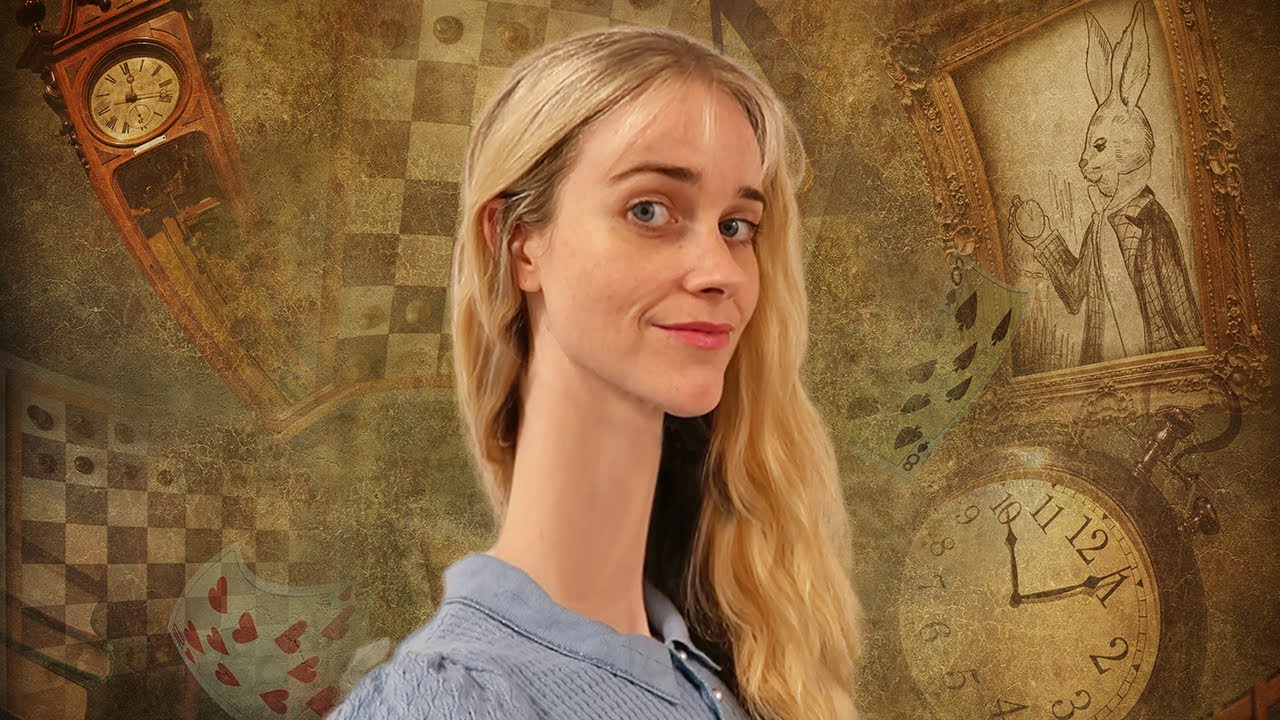# MathematicsMathematics

#### How to Make Railway Timetables with Graphs

Hannah Fry discusses the mathematics behind Train Graphs. ,Data Science Mathematics Python

#### Introduction to Monte Carlo Methods in Python

This video includes a basic tutorial in Monte Carlo simulation techniques in python, along with a few examples. CodeMathematics

#### How Imaginary Numbers Were Invented

Imaginary numbers come up in the most unlikely places. Quite impressive given that they are “impossible.” But how did math go from reflecting and describing reality to the abstract field we tend to think of it today. Veritasium explains.Data Science Mathematics

#### Practical Statistics for Data Scientists – Exploratory Data Analysis

This is an overview of Chapter 1 of Practical Statistics for Data Scientists. Shashank Kalanithi goes over the first couple of chapters of this book because the later chapters cover similar materials from my Hands on Machine Learning book overview videos.Mathematics

#### Jo Boaler on How to Learn Math | Lex Fridman Podcast #226

Given the increasing importance of mathematics in the world, it may be as crucial to success as literacy. Jo Boaler is a professor of mathematics education at Stanford and the co-founder of youcubed. In this video, she sits down with Lex Fridman to talk about learning math. OUTLINE: 0:00 – Introduction 0:23 – What is […]Mathematics

#### The Lightning Algorithm

Here’s a bit of math to get your Monday started. This time Matt Henderson is making lightning in mazes.Mathematics

#### Is Math Real?

Sabine Hossenfelder discusses whether or not math is real.Mathematics

#### A Pattern In Primes

Peter Pike explains while prime numbers seem to show up randomly on the number line, they are built on very specific patterns.Mathematics

#### Hidden Math in Alice in Wonderland

Tibees explains the math inside of a classic story.